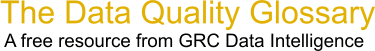Choose Index below for a list of all words and phrases defined in this glossary.

# Standard Deviation Classification

index | Index

## Standard Deviation Classification - definition(s)

standard deviation classification - [statistics] A data classification method that finds the mean value, then places class breaks above and below the mean at intervals of either .25, .5, or 1 standard deviation until all the data values are contained within the classes. Values that are beyond three standard deviations from the mean are aggregated into two classes, greater than three standard deviations above the mean and less than three standard deviations below the mean.

[Category=Geospatial ]

Source: esri, 29 August 2012 08:17:02, http://support.esri.com/en/knowledgebase/GISDictionary/term/abbreviationData Quality Glossary.  A free resource from GRC Data Intelligence. For comments, questions or feedback: dqglossary@grcdi.nl